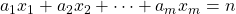# A formula for the number of non-negative integer solutions of a1x1 + a2x2 + ··· + amxm = n in terms of the partial Bell polynomials

Sumit Kumar Jha
Notes on Number Theory and Discrete Mathematics
Print ISSN 1310–5132, Online ISSN 2367–8275
Volume 27, 2021, Number 2, Pages 64—69
DOI: 10.7546/nntdm.2021.27.2.64-69

## Details

### Authors and affiliations

Sumit Kumar JhaInternational Institute of Information Technology

### Abstract

We derive a formula for the number of non-negative integer solutions of the equationin terms of the partial Bell polynomials via the Faa di Bruno’s formula.

### Keywords

• Linear Diophantine equation
• Generating function

• 11D45
• 05A15

### References

1. Komatsu, T. (2003). On the number of solutions of the Diophantine equation of Frobenius – General case. Mathematical Communications, 8, 195–206.
2. Tripathi, A. (2000). The number of solutions to ax + by = n. The Fibonacci Quarterly, 38, 290–293.
3. Binner, D. S. (2020). Number of non-negative integer solutions of the equation
ax + by + cz = n and its relation with quadratic residues. Journal of Integer Sequences, 23, Article 20.6.5.
4. Comtet, L. (1974). Advanced Combinatorics: The Art of Finite and Infinite Expansions, D. Reidel Publishing Co., Dordrecht.
5. Charalambides, Ch. A. (2002). Enumerative Combinatorics, CRC Press.
6. Eger, S. (2016). Identities for partial Bell polynomials derived from identities for weighted integer compositions. Aequationes mathematicae, 90, 299–306.
7. SymPy: a Python library for symbolic mathematics. Tool available at https://docs.sympy.org/latest/modules/functions/combinatorial.html.

## Cite this paper

Jha, S. K. (2021). A formula for the number of non-negative integer solutions of a1x1 + a2x2 + ··· + amxm = n in terms of the partial Bell polynomials. Notes on Number Theory and Discrete Mathematics, 27(2), 64-69, doi: 10.7546/nntdm.2021.27.2.64-69.